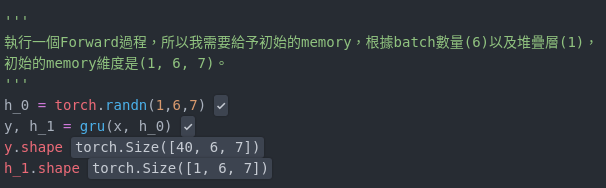# Initial hidden state of GRU when testing the real data

I try to understand how GRU work in the model.
I think I known how it work in the forward process.
Here is my example for demo forward follow blow.For each input data, I need to initialize a hidden state.
In training process, I can randomly initialize it.
After finishing training process, if I want to test a real data, how to determine the initial hidden state?
Still by randomly choose? Or I make some mistake about forward of GRU?

Use the same method for both train and test (random initialization in this case).

The same input data, different initial state, will get the different result, is it normal?

Can you elaborate on what you exactly mean?

1. Did you mean that the same model after training is used with different initial states?
2. Did you mean training with different initial states?